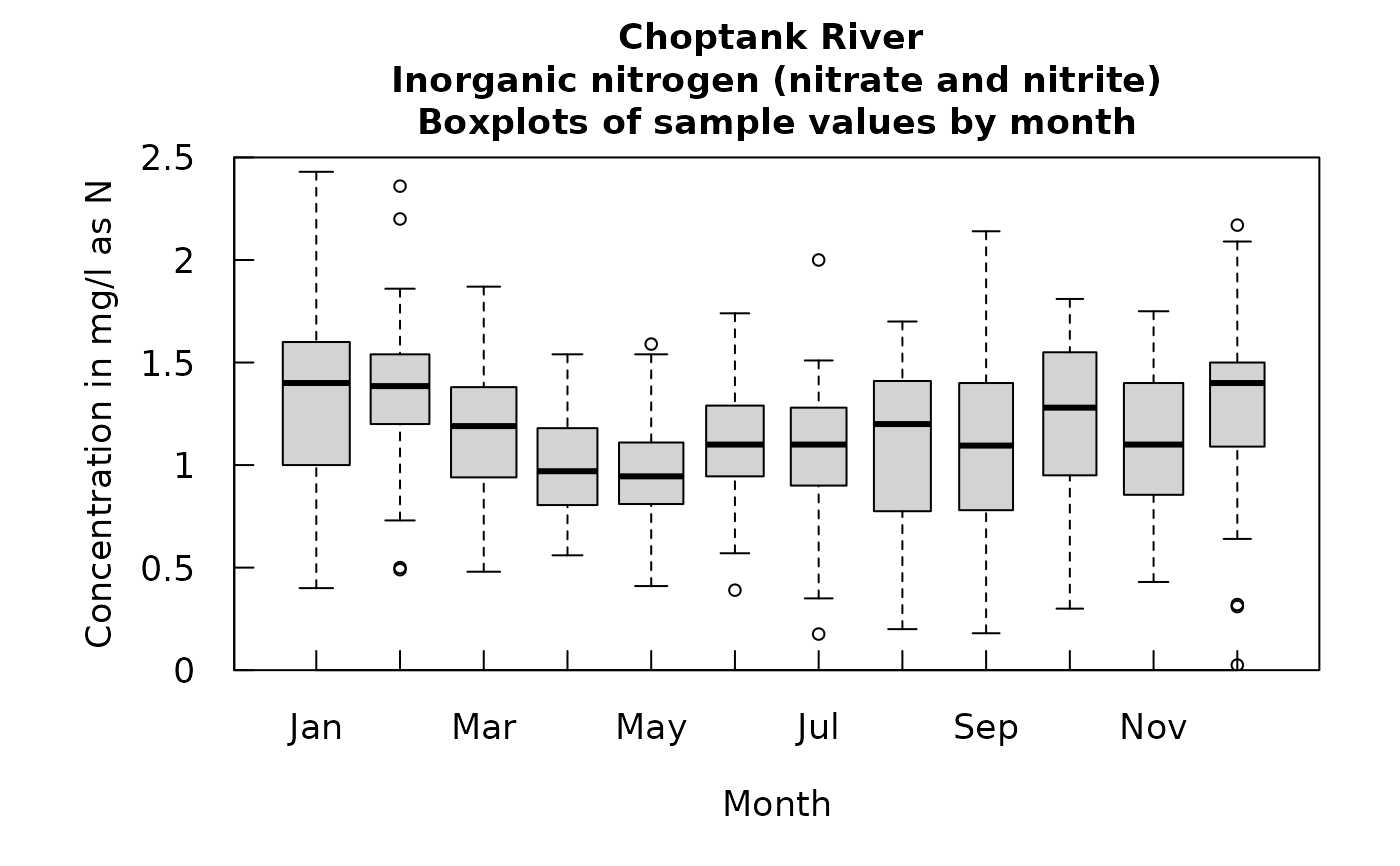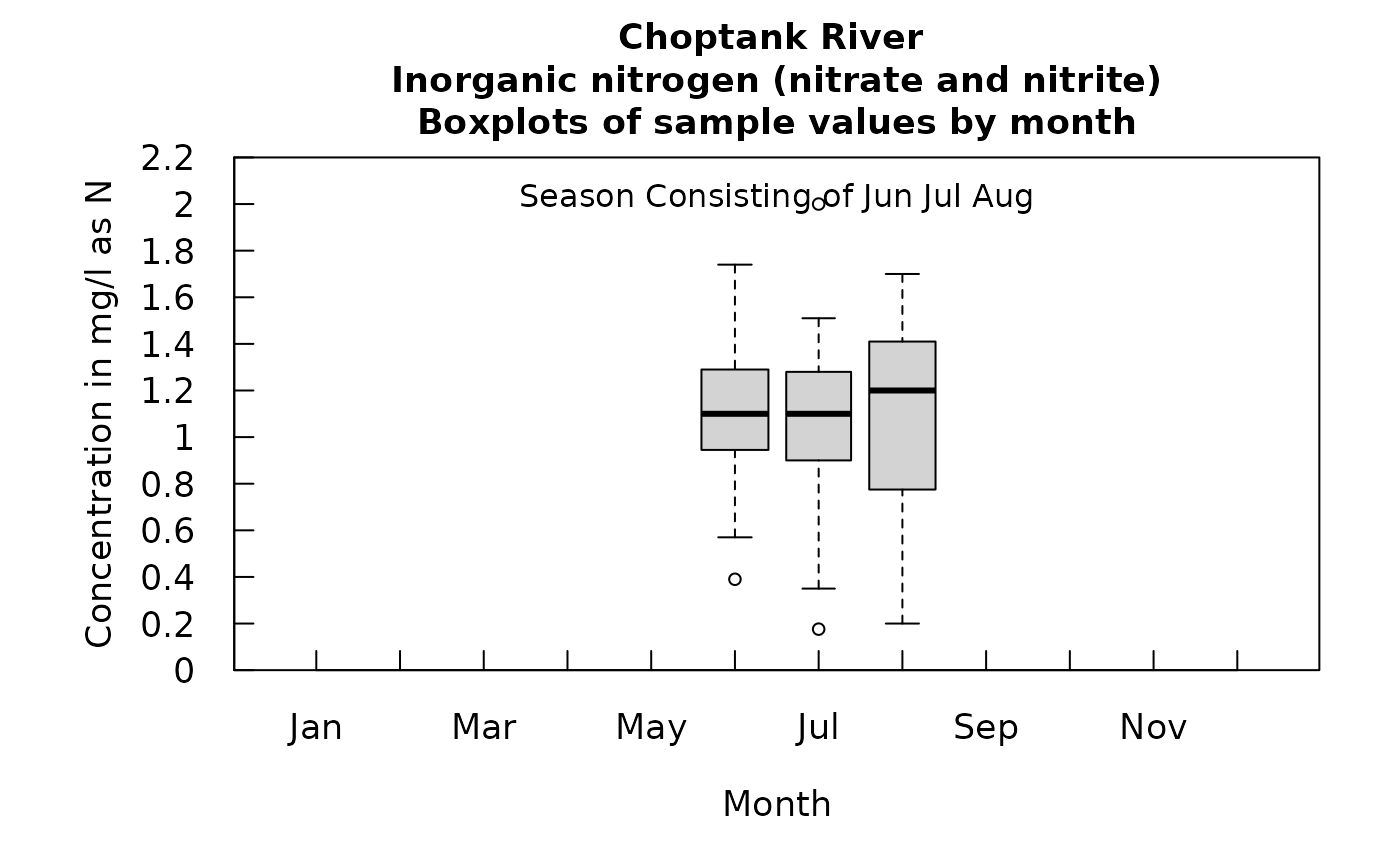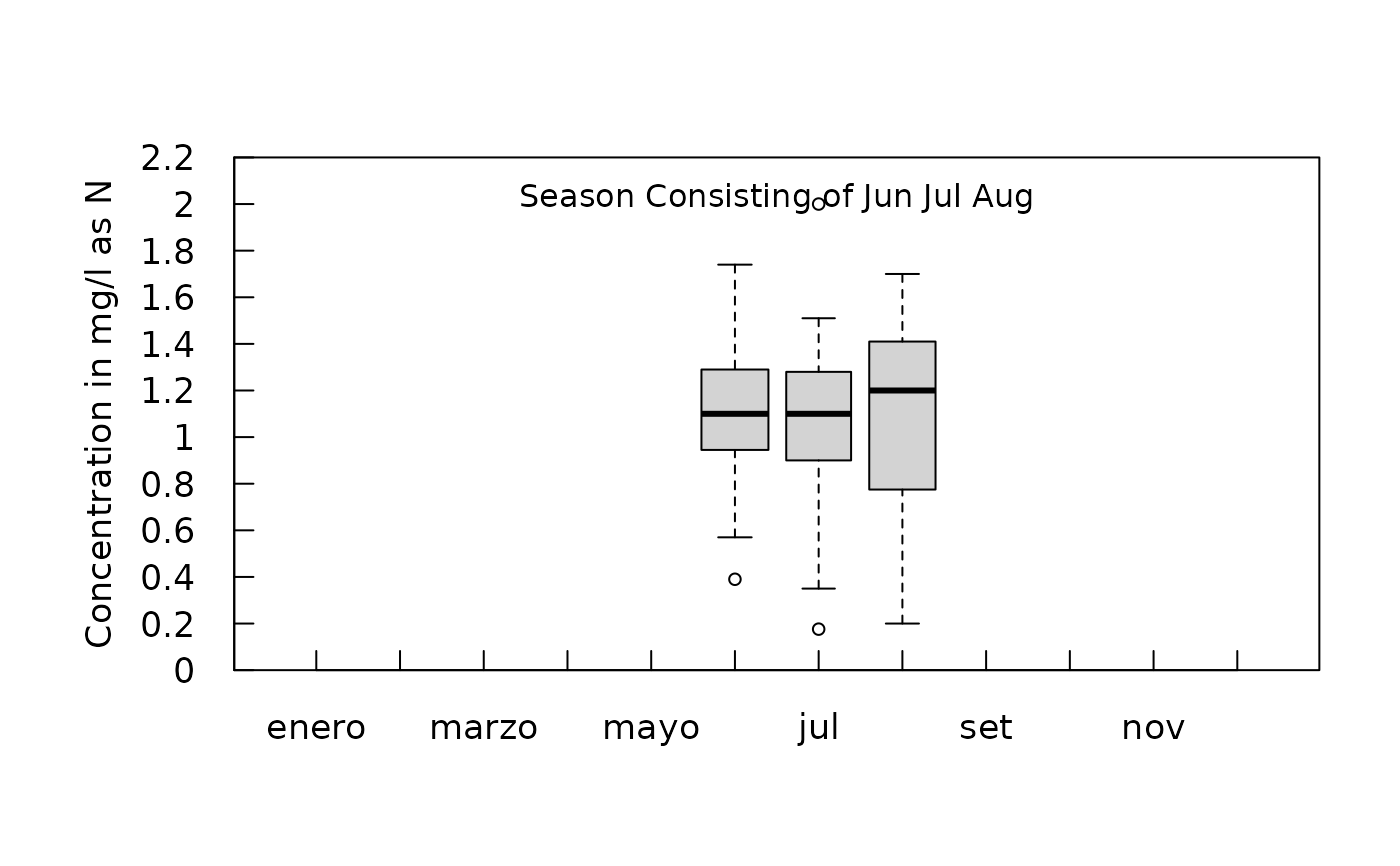Data come from named list, which contains a Sample dataframe with the sample data, and an INFO dataframe with metadata.

Box widths are proportional to the square root of the number of samples in the month.

Although there are a lot of optional arguments to this function, most are set to a logical default.

## Usage

boxConcMonth(eList, printTitle = TRUE, cex = 0.8, cex.axis = 1.1,
cex.main = 1.1, las = 1, logScale = FALSE, tcl = 0.5,
tinyPlot = FALSE, customPar = FALSE, showYLabels = TRUE, concLab = 1,
showXLabels = TRUE, showXAxis = TRUE, showYAxis = TRUE, monthLab = 1,
...)

## Arguments

eList

named list with at least the Sample and INFO dataframes

printTitle

logical variable if TRUE title is printed, if FALSE not printed (this is best for a multi-plot figure)

cex

numerical value giving the amount by which plotting symbols should be magnified

cex.axis

magnification to be used for axis annotation relative to the current setting of cex

cex.main

magnification to be used for main titles relative to the current setting of cex

las

numeric in 0,1,2,3; the style of axis labels, see ?par

logScale

logical if TRUE y plotted in log axis

tcl

number defaults to 0.5, specifies length of tick marks as fraction of height of a line of text

tinyPlot

logical variable, if TRUE plot is designed to be plotted small as part of a multi-plot figure, default is FALSE.

customPar

logical defaults to FALSE. If TRUE, par() should be set by user before calling this function

showYLabels

logical defaults to TRUE. If FALSE, the y axis label is not plotted

concLab

object of concUnit class, or numeric represented the short code, or character representing the descriptive name. By default, this argument sets concentration labels to use either Concentration or Conc (for tiny plots). Units are taken from the eList$INFO$param.units. To use any other words than "Concentration" see vignette(topic = "units", package = "EGRET").

showXLabels

logical defaults to TRUE. If FALSE, the x axis label is not plotted

showXAxis

logical defaults to TRUE. If FALSE, the x axis is not plotted

showYAxis

logical defaults to TRUE. If FALSE, the y axis is not plotted

monthLab

object of monthLabel class, or numeric represented the short code, or character representing the descriptive name.

...

arbitrary graphical parameters that will be passed to genericEGRETDotPlot function (see ?par for options)

boxplot

## Examples

eList <- Choptank_eList
# Water year:
boxConcMonth(eList)# Graphs consisting of Jun-Aug
eList <- setPA(eList, paStart=6,paLong=3)
boxConcMonth(eList)spanish_month <- new("monthLabel",
monthAbbrev = c("enero",  "feb",   "marzo", "abr",
"mayo",  "jun",  "jul", "agosto", "set",
"oct",  "nov", "dic"),
monthFull = c("enero",  "febrero",   "marzo", "abril",
"mayo",  "junio",  "julio", "agosto", "septiembre",
"octubre",  "noviembre", "diciembre"),
monthSingle = c("E", "F", "M", "A", "M", "J", "J",
"A", "S", "O", "N", "D"))
boxConcMonth(eList, monthLab = spanish_month,
showXLabels = FALSE, printTitle = FALSE)